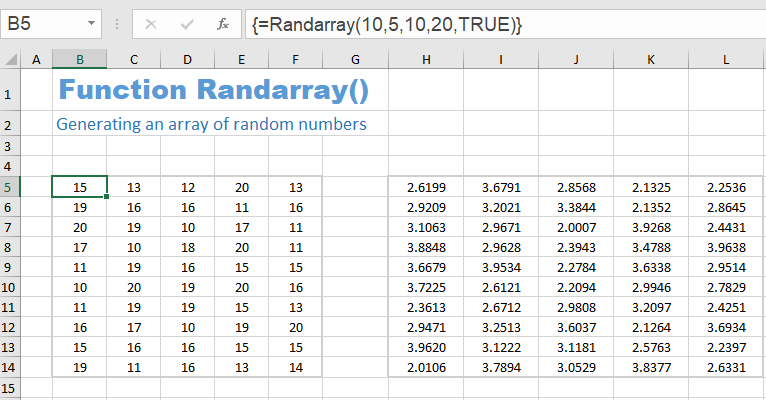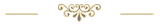Last update: 08.06.2022Download the Excel file with the VBA code of the functions SORT, SORTBY, FILTER, XLOOKUP, XMATCH, UNIQUE, SEQUENCE, RANDARRAY and all examples shown on these web pages:
(Version 3.4 - Last update: 2022-03-15)

In Excel 365 and from Excel 2021, Microsoft implemented the long-missing cell functions SORT, SORTBY, FILTER, XLOOKUP and others. However, they were not retrofitted for older versions of Excel, so that all users from Excel 2007 up to and including Excel 2019 had to do without them.

There is now a solution for users of older versions of Excel (since the end of January 2022).

With the help of VBA so-called UDFs (user defined functions) were implemented that have exactly the same parameter lists as the corresponding Microsoft functions.

In cases where the functions provided here return multiple values, their input must be terminated with CTRL-SHIFT-ENTER. Before entering the formula, an area must be selected on the spreadsheet that contains the returned data - as is usual with the so-called CSE array formulas (CSE = Control Shift Enter).

This tutorial provides a basic and detailed introduction to array formulas.

With Function names of UDFs it doesn't matter whether the letters are written in upper or lower case.

For instructions on intergrating the functions in your own Excel file, see ▸Integrating the functions.

### RANDARRAY function

The RANDARRAY function allows you to generate an array of random numbers. You can choose the size of the target area (number of lines and columns) as well as the lower and upper limit of the random numbers and you can specify whether they should be integer values or decimal numbers.

#### Syntax

= Randarray ( [rows], [columns], [min], [max], [integer] )

Parameter Explanation
rows
(optional)
Number of rows in the result array
(default: 1)
columns
(optional)
Number of rows in the result array
(default: 1)
min
(optional)
Lower limit of the number range
(default: 0)
max
(optional)
Upper limit of the number range
(default: 1)
integer
(optional)
FALSE  for decimal numbers (default)
TRUE   for integer numbers

The Randarray function has the same behavior as the Excel 365 function RANDARRAY.
https://support.microsoft.com/en-us/office/randarray-function-21261e55-3bec-4885-86a6-8b0a47fd4d33

#### Example

A 10 ✕ 5 array of random integer numbers in the range 10 to 20 is generated and displayed in the selected target area. Before entering this formula, it makes sense to select an area of 10 rows and 5 columns.

The formula is:  = Randarray (10, 5, 10, 20, TRUE)The formula of the second random array is:
= Randarray (10, 5, 10, 20).
They are decimal numbers with a lower bound of 10 and an upper bound of 20. Unlike the integer random numbers, the upper bound of 20 is excluded here (and the lower bound is extremely unlikely).Sharing knowledge is the future of mankind